## Plots in R

The function plot() is called to generate a plot in R. This function has many arguments. We will look at the most common in these notes.

### Points

The first arguments we must give are the $$x$$ ordinates and the $$y$$ ordinates of the points to be plotted. These are held as vectors and passed to plot(). To illustrate, suppose we want to plot the two points:

\begin{align*} & (1, 4) \\ & (5, 11) \end{align*}

Then we should define X <- c(1, 5) to hold the $$x$$ ordinates and Y <- c(4, 11) to hold the $$y$$ ordinates.

X <- c(1, 5)
Y <- c(4, 11)
plot(X,Y)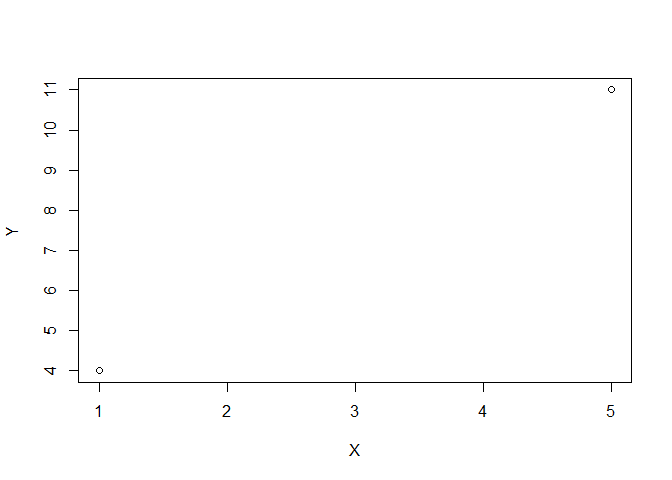Note, the ordering matters: X <- c(1, 5) but with Y <- c(11, 4) gives a different plot:

X <- c(1, 5)
Y <- c(11, 4)
plot(X,Y)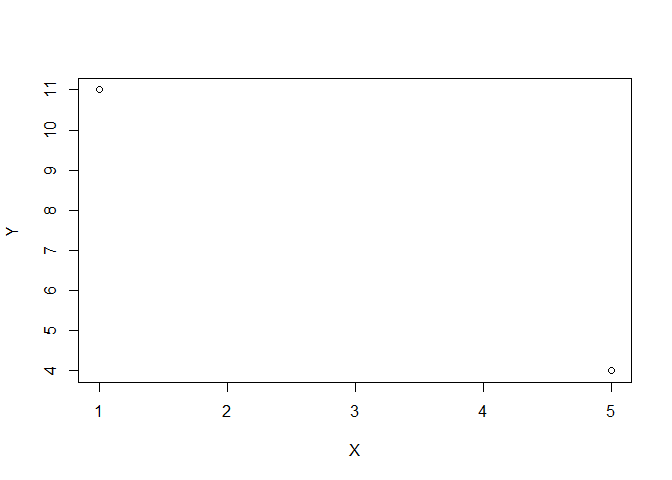Here’s how we can begin to plot the graph of a function:

X <- seq(-5, 5, by = 0.1)
Y <- X^2
plot(X,Y)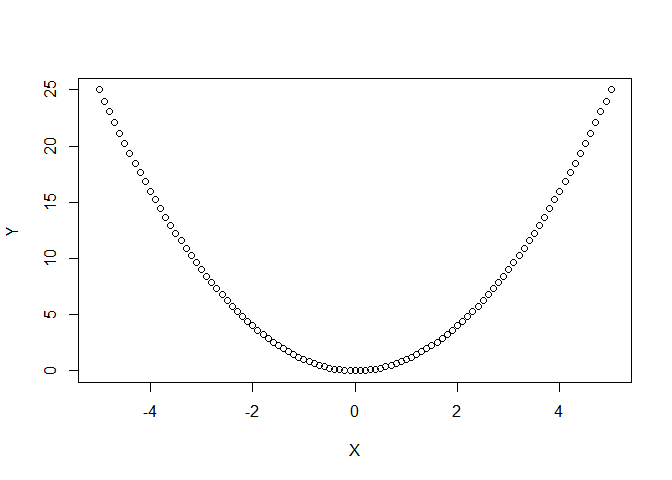X <- seq(-5, 5, by = 0.1)
Y <- sin(X)
plot(X,Y)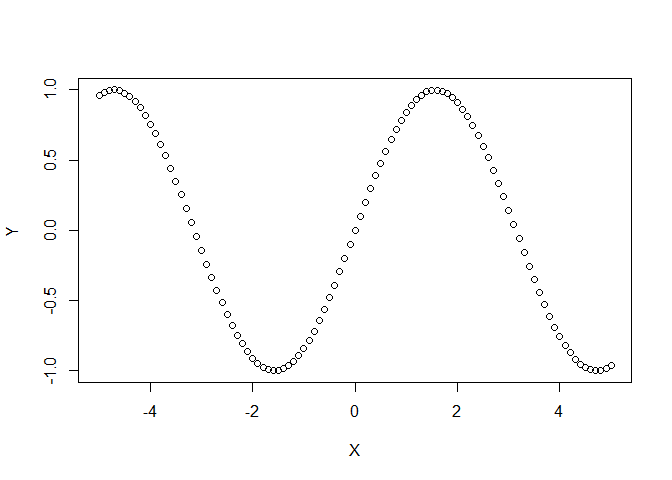### Point Colour

The argument col can be used to specify the colour of the points in different ways as shown in the following examples.

The colour can be given as text in quotations. R knows the names of many colours. You can easily find a list of such colour names online.

X <- seq(-5, 5, by = 0.1)
Y <- sin(X)

#notice the "col" argument in the plot function:
plot(X,Y, col = "blue")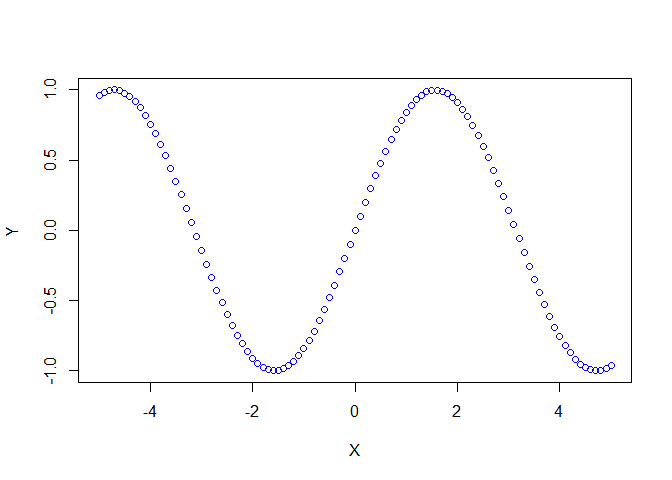The colour can be specified as HEX code as in the following example:

X <- seq(-5, 5, by = 0.1)
Y <- sin(X)

#notice the hex code is placed between quotation marks:
plot(X,Y, col = "#B22222")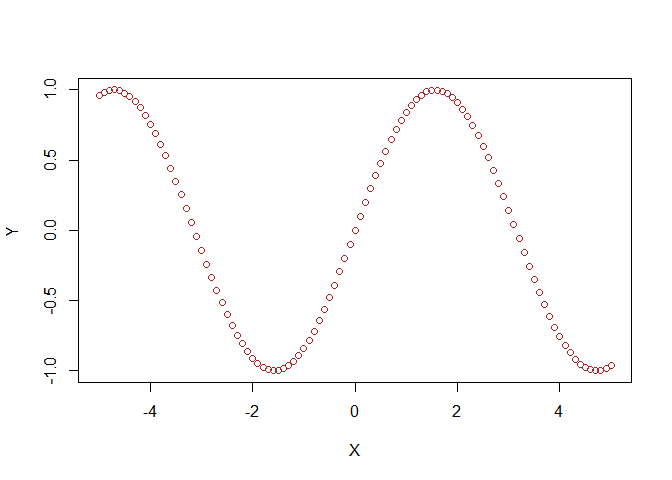The colour can be cycled through a vector of colours:

X <- seq(-5, 5, by = 0.1)
Y <- sin(X)

colours <- c("red", "pink", "purple", "blue")
plot(X,Y, col = colours)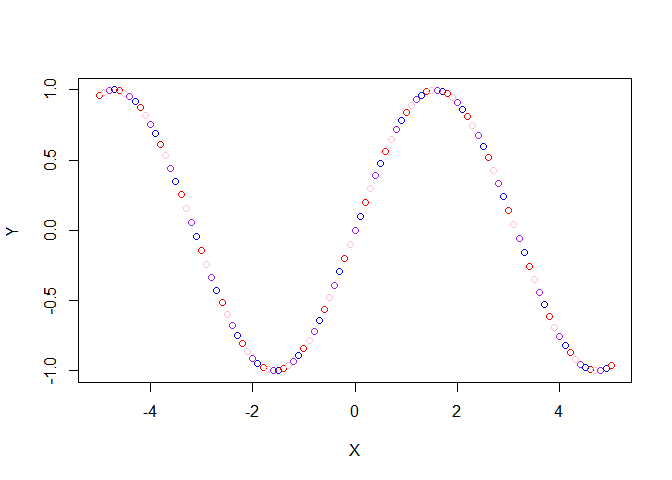You can even create a cool rainbow effect using a function called colorRampPalette():

X <- seq(-5, 5, by = 0.1)
Y <- sin(X)

colours <- colorRampPalette(c("red", "pink", "purple", "blue"))(40)
plot(X,Y, col = colours)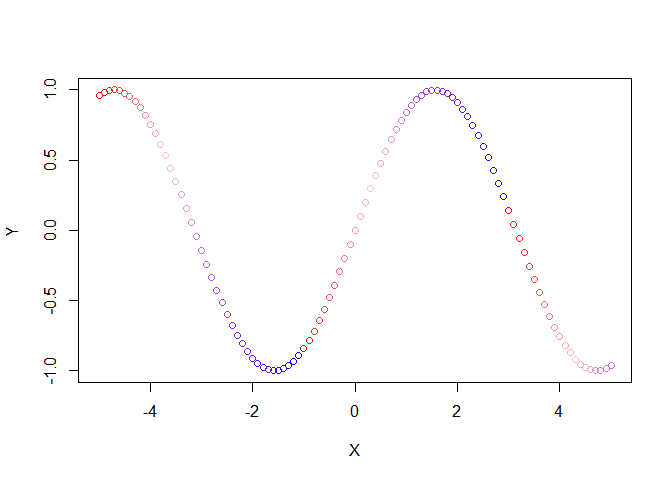The number specified in brackets controls the length of the colour cycle. Note the difference in the following example.

X <- seq(-5, 5, by = 0.1)
Y <- sin(X)

colours <- colorRampPalette(c("red", "pink", "purple", "blue"))(100)
plot(X,Y, col = colours)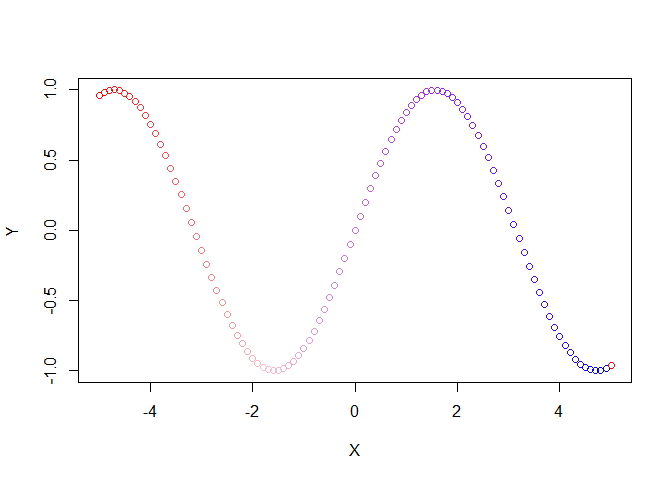### Point Character and Size

The arguments pch and cex can be added to control the point character and size respectively.

Here are some examples:

X <- seq(0, 10, by = 0.1)
Y <- sin(X)

plot(X,Y, pch = 16, cex = 0.5)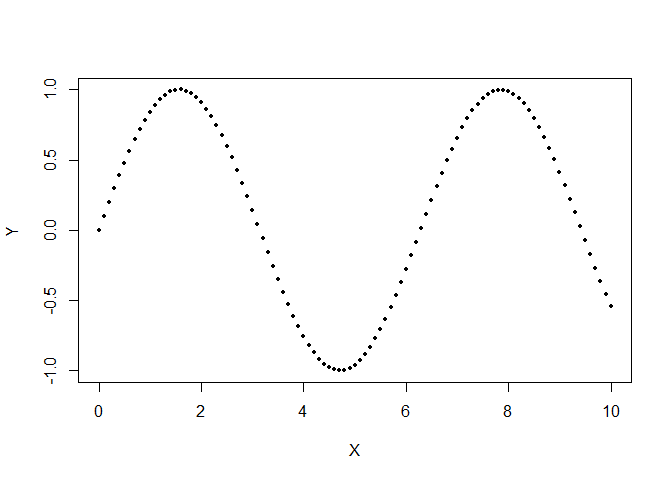plot(X,Y, pch = "#", cex = 1)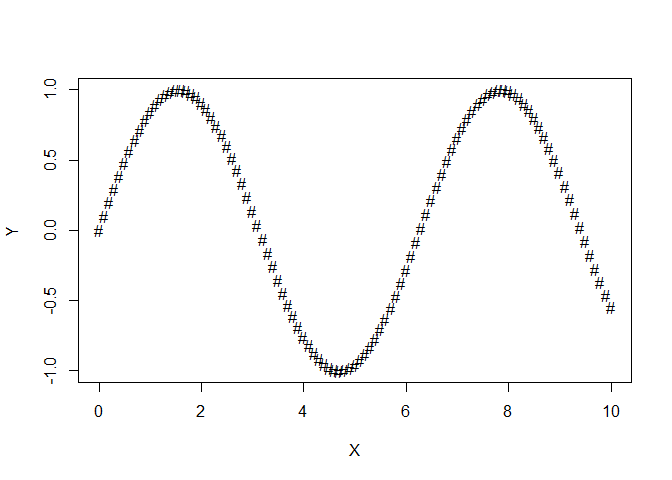plot(X,Y, pch = 25, cex = 1.2, col = colours)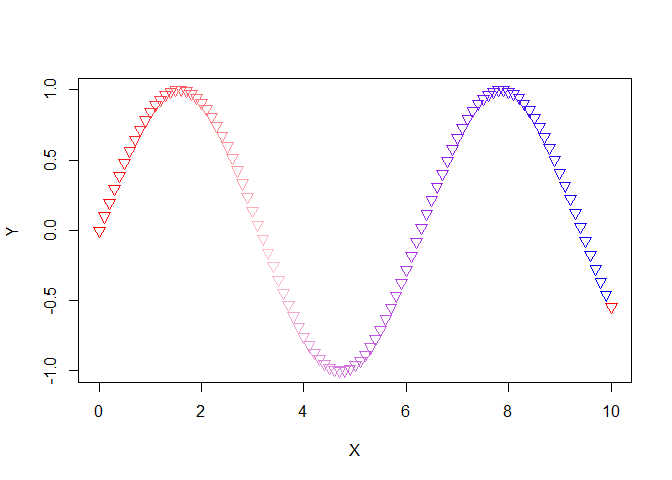plot(X,Y, pch = 1, cex = X)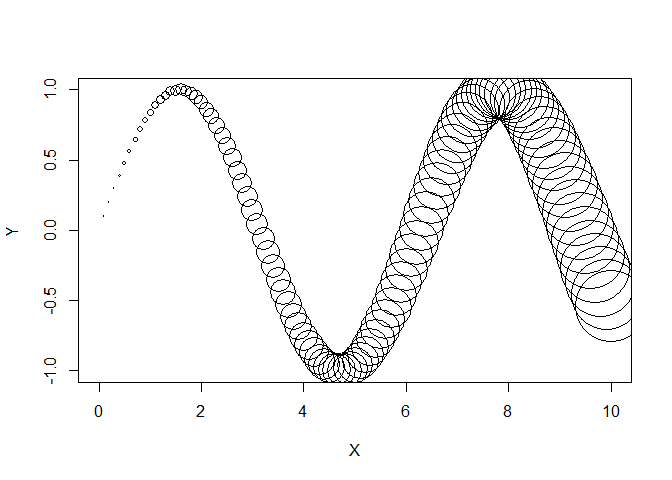Search online for different pch values or just experiment for yourself! Notice in the last example that the point size was controlled by X so that the points plotted increased in size as $$x$$ increased along the horizontal axis.

### Point and/or Line

Add the argument type in the plot function. It can be assigned p for point, l for line or b for both. The following examples illustrate:

X <- seq(0, 10, by = 0.2)
Y <- sin(X)
plot(X,Y, pch = 16, cex = 1.2, col = colours, type ="p")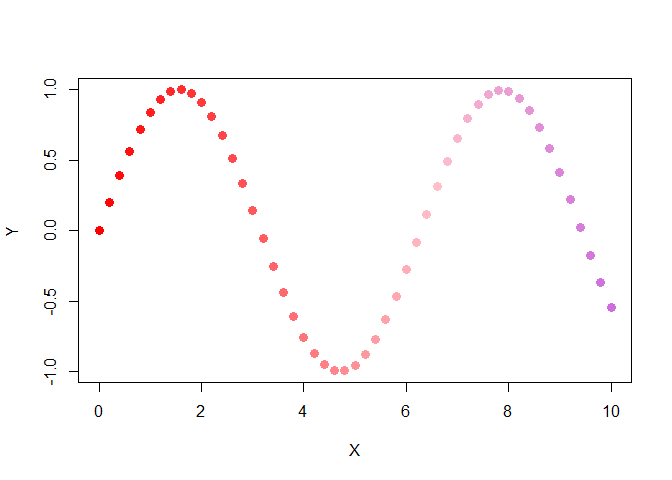X <- seq(0, 10, by = 0.01)
Y <- sin(X)
plot(X,Y, pch = 16, cex = 1.2, col = "black", type ="l")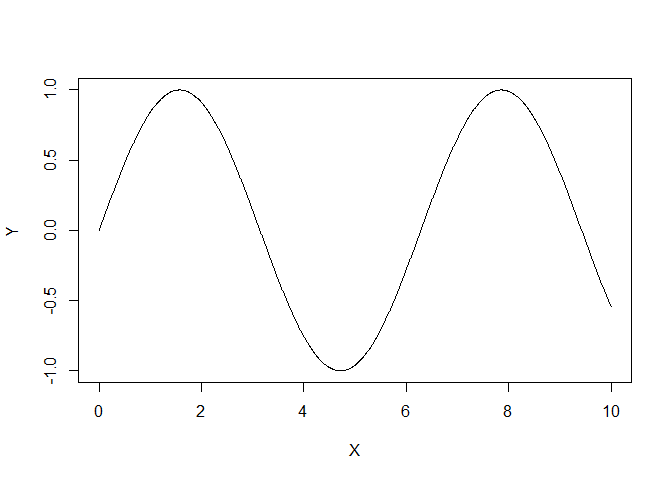X <- seq(0, 10, by = 0.2)
Y <- sin(X)
plot(X,Y, pch = 16, cex = 1.2, col = colours, type ="b")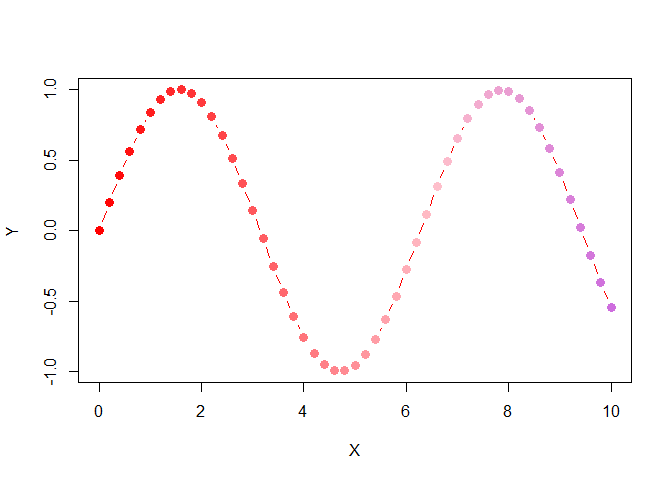### Plot Limits

We can specify the size of the plot by giving limits for the $$x$$ and $$y$$ axes. We do so by addling xlim and ylim as in the following example:

X <- seq(-4, 4, by = 0.2)
Y <- tan(X)
plot(X,Y, type = "l", col = "blue", xlim = c(-4,4), ylim = c(-10, 10))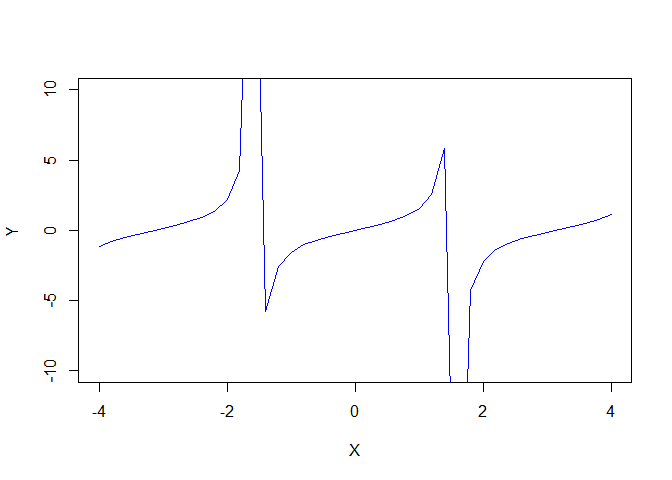This plot of $$\tan(x)$$ is obviously not very good! One problem has been caused by specifying type as "l". This forces the plot to draw a strange looking line joining points across asymptotes. Let’s try again:

X <- seq(-4, 4, by = 0.0001)
Y <- tan(X)
plot(X,Y, type = "p", pch = ".", col = "blue", xlim = c(-4,4), ylim = c(-10, 10))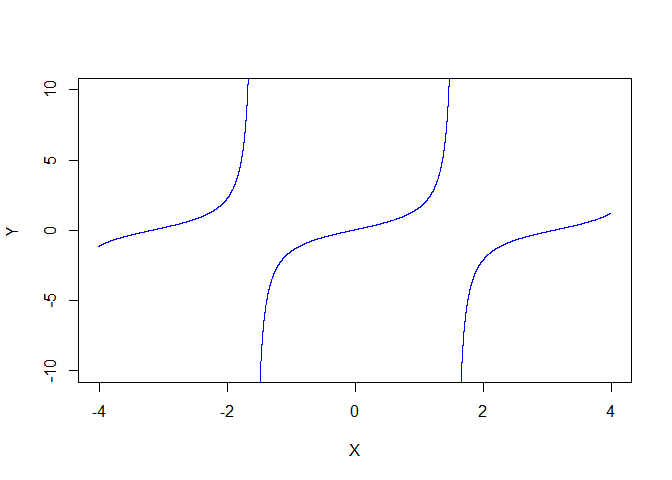In the second plot we increased the “resolution” with by = 0.0001 and plotted points only using pch = "." and type = "p".

### Plot Background and adding points and lines

If you like, you can add a coloured background to your plots.

#first create a "null plot". Also note the arguments to add labels to the axes.
plot(NULL, xlim = c(-5,5), ylim = c(-1.5, 1.5), xlab = "x axis", ylab = "y axis")

#the following rather cumbersome code adds a coloured rectangle to the blank plot.
#The size of the rectangle automatically fits the plot size.
rect(par("usr"), par("usr"), par("usr"), par("usr"), col = "gray10")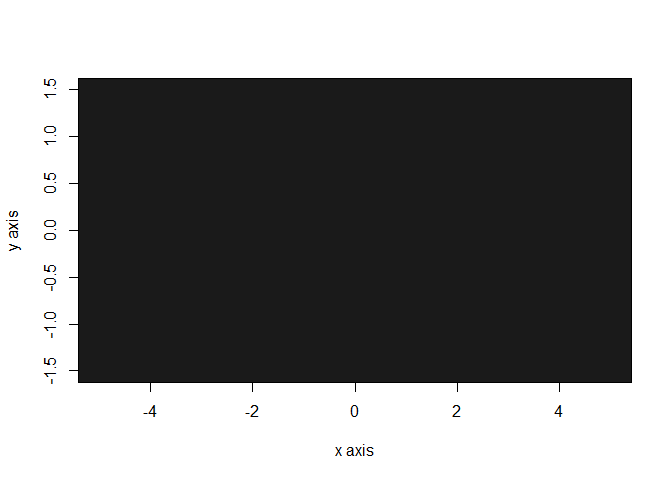Now let’s add the graph of $$\sin(x)$$ onto this dark grey background. We cannot call plot() to do this since a freash plot will be created. Instead, we use the points() function to add points onto a pre-existing plot:

plot(NULL, xlim = c(-5,5), ylim = c(-1.5, 1.5), xlab = "x axis", ylab = "y axis")

rect(par("usr"), par("usr"), par("usr"), par("usr"), col = "gray10")

X <- seq(-5, 5, by = 0.1)
Y <- sin(X)
colours = colorRampPalette(c("red", "pink", "blue", "purple"))(40)

points(X,Y, pch = 16, cex = 1.2, col = colours)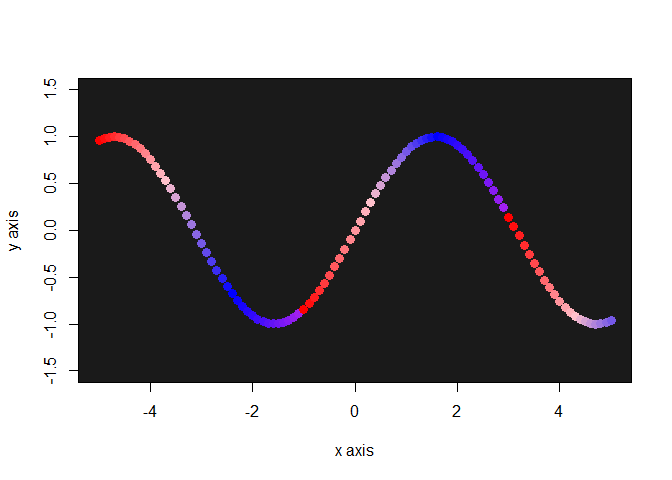Lines can be added to pre-existing plots also. Suppose we want to add the asymptotes to the earlier plot of $$\tan(x)$$. Then we can do so as follows:

X <- seq(-4, 4, by = 0.0001)
Y <- tan(X)
plot(X,Y, type = "p", pch = ".", col = "blue", xlim = c(-4,4), ylim = c(-10, 10))

#note the "lwd" argument used to alter the line width:
lines(c(-pi/2, -pi/2), c(-10, 10), lwd = 1.5, col = "darkred")
lines(c(pi/2, pi/2), c(-10, 10), lwd = 1.5, col = "darkred")

lines(c(-4,4), c(0,0), col = "black", lwd = 2)
lines(c(0,0), c(-10,10), col = "black", lwd = 2)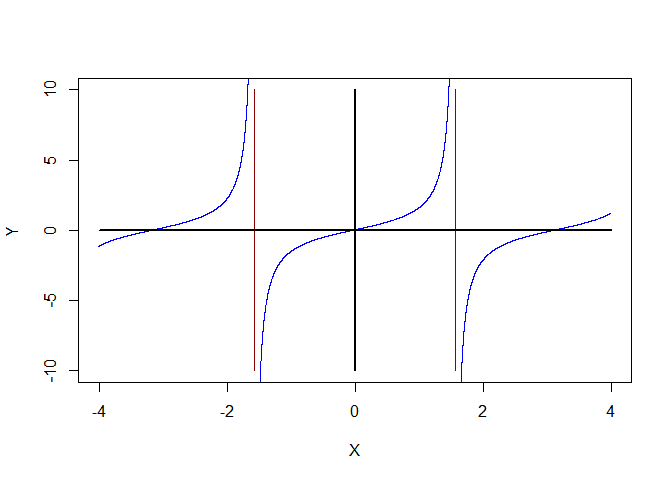### Legend

Finally, a legend can be added to your plot. First, let’s create a plot showing two functions:

\begin{align*} y_1 & = x^{3} - 5x^2 +4x +1 \\ y_2 & = \sin(x) \end{align*}
X <- seq(-5, 5, by = 0.001)
Y1 <- X^3 - 5*X^2 + 4*X + 1
Y2 <- sin(X)

plot(X,Y1, xlim = c(-5, 5), ylim = c(-10, 10), col = "red", type = "l", lwd = 1)
lines(X,Y2, lwd = 1, col = "blue")

#the first two numbers position the legend
#the "legend" argument is a character vector
#col defines the legend line colour which should match the lines described

legend(-4, 10, legend = c("x^3 - 5x^2 + 4x + 1", "sin(x)"), col = c("red", "blue"), lty = 1)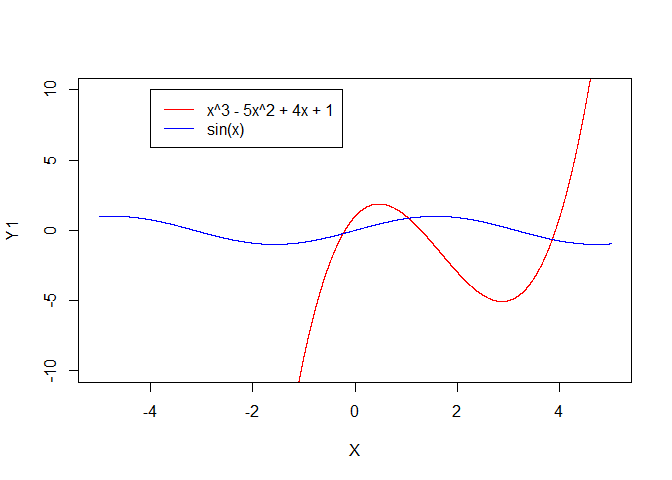There are many possibilities for adjusting the legend. Search online for whatever you need!